# Problem of two honest buddies

Hello Everyone,

Skip this para If you want to you dive into the problem. Some of you guys might be pushed (as I am going to mention you in comment after writing this) or you might be visiting this discussion on your own. In both circumstances my colossal thanks to you guys Because I was wondering about the solution of this problem and was getting different explanations from every part of internet most of them were wrong so now I might get the answer.

  PROBLEM IS HERE :- Problem is as follows two plane mirrors are placed at an angle of 50 degrees to each other and an object(point sized) is placed symmetrically between them You has to find the no. of images formed.

• You use any formula provide it. I used a formula it gives answer which differs from my graphical solution. [I prefer you provide your solution with some graphical representation like an image I am providing below.]

Below are two images one is an example for an angle between mirrors 90 degrees other is for 50 degrees as asked in question.

• Better If open images in new tab.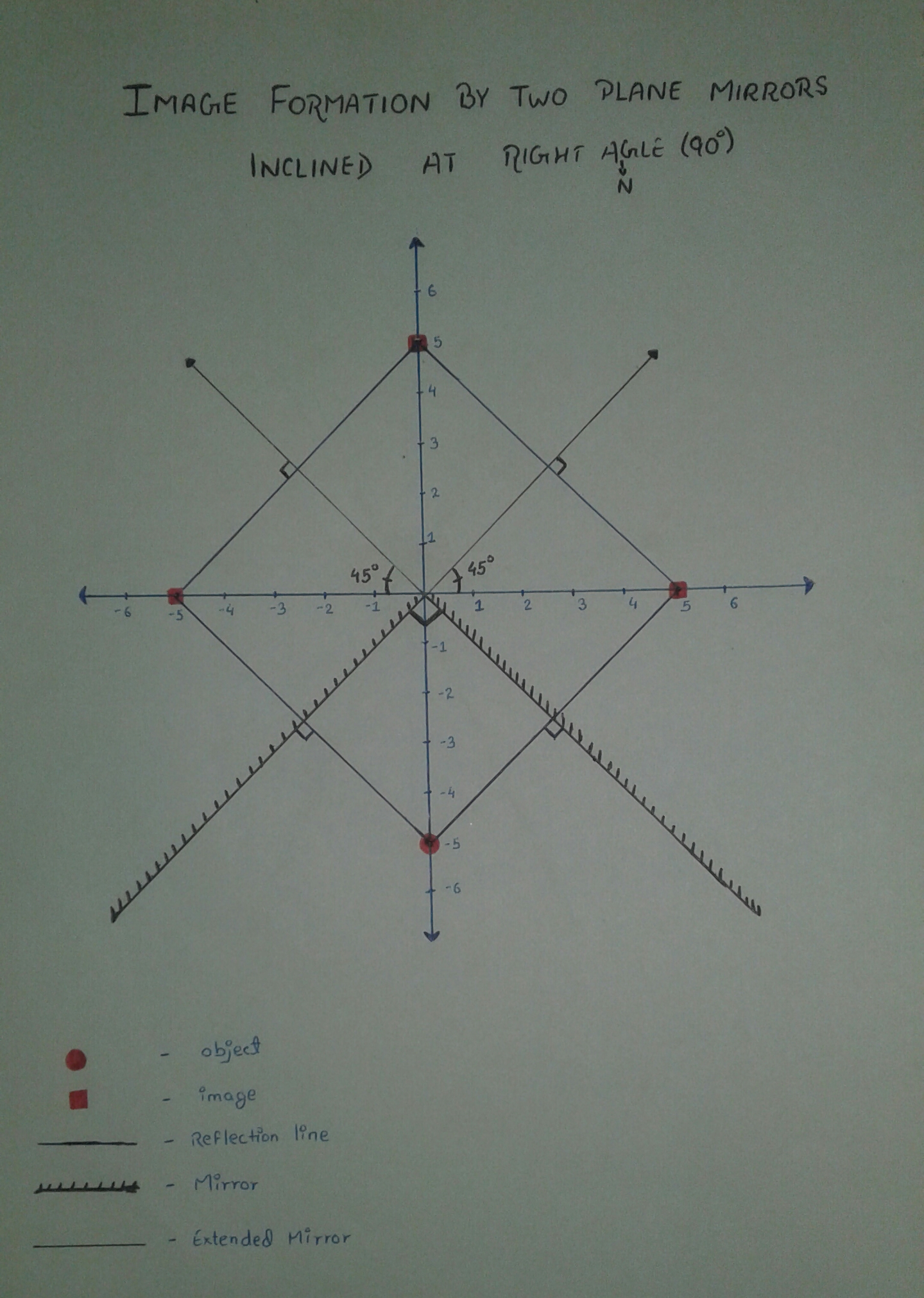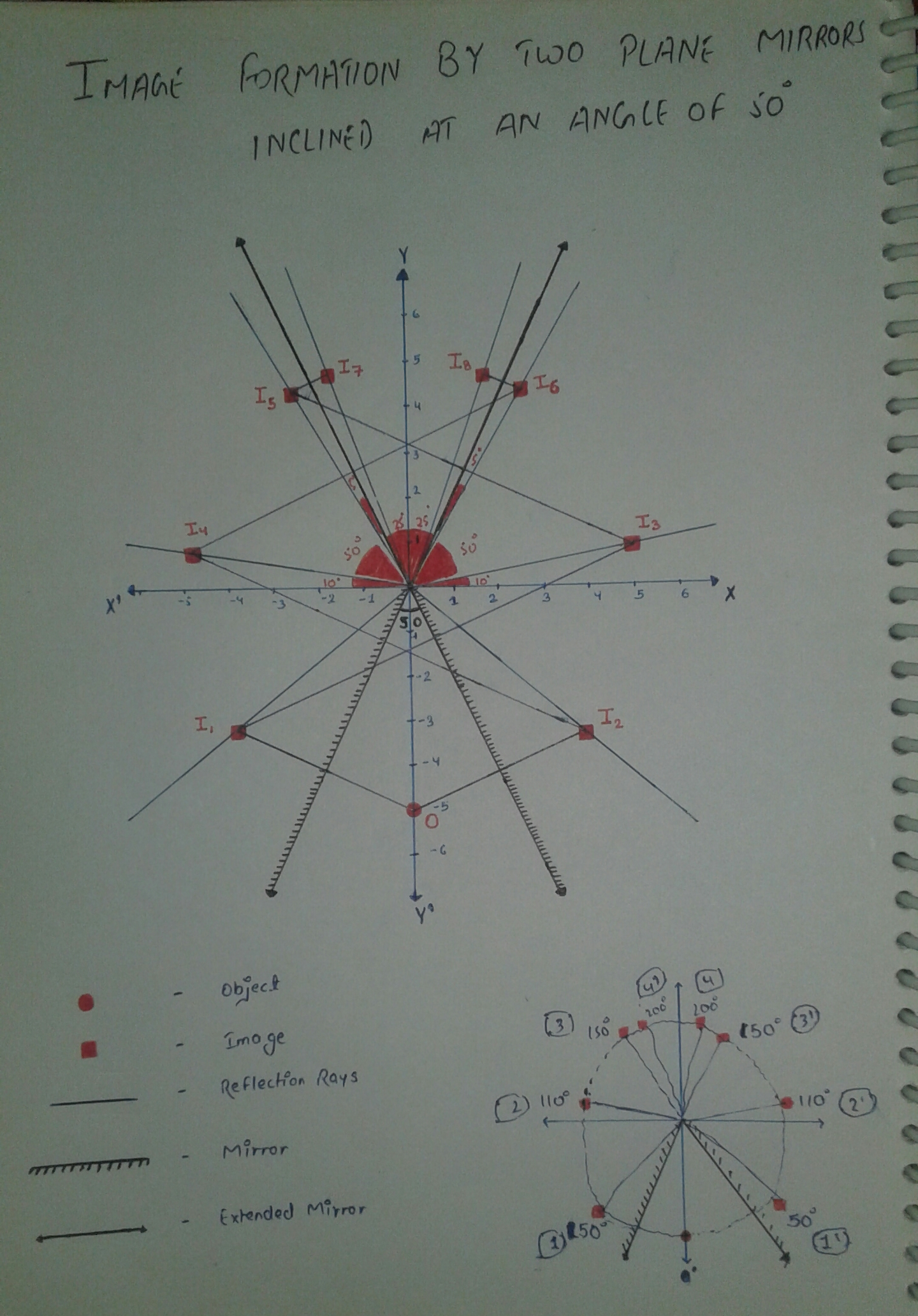Edited : I find out some of you have problem in understanding the images so a short explanation here ==>

            You can see mirrors in third and fourth quadrant and object is at -ve y axes at a distance of -5 from origin them first to images are formed by two mirrors Ɪ1 and Ɪ2 then what happens is image Ɪ2 works as a virtual object for left side mirror and Ɪ1 works as a virtual object for right side mirror and then It happens again and again. This stops when any images go beyond the mirror(extended) because then Image cannot work as virtual object. Below are given virtual object/object and their images.


O ---> Ɪ1 , Ɪ2

Ɪ1 ---> Ɪ3 , Ɪ2 ---> Ɪ4

Ɪ3 ---> Ɪ5 , Ɪ4 ---> Ɪ6

Ɪ5 ---> Ɪ7 , Ɪ6 ---> Ɪ8

   Laws used in Graph-

• line joining image and object/virtual object is perpendicular to mirror.
• Distance of image is equal to Distance of object from mirror/origin.

                                                         Thank you very much for visiting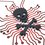Note by Vikram Karki
1 year, 1 month ago

This discussion board is a place to discuss our Daily Challenges and the math and science related to those challenges. Explanations are more than just a solution — they should explain the steps and thinking strategies that you used to obtain the solution. Comments should further the discussion of math and science.

When posting on Brilliant:

• Use the emojis to react to an explanation, whether you're congratulating a job well done , or just really confused .
• Ask specific questions about the challenge or the steps in somebody's explanation. Well-posed questions can add a lot to the discussion, but posting "I don't understand!" doesn't help anyone.
• Try to contribute something new to the discussion, whether it is an extension, generalization or other idea related to the challenge.

MarkdownAppears as
*italics* or _italics_ italics
**bold** or __bold__ bold
- bulleted- list
• bulleted
• list
1. numbered2. list
1. numbered
2. list
Note: you must add a full line of space before and after lists for them to show up correctly
paragraph 1paragraph 2

paragraph 1

paragraph 2

[example link](https://brilliant.org)example link
> This is a quote
This is a quote
    # I indented these lines
# 4 spaces, and now they show
# up as a code block.

print "hello world"
# I indented these lines
# 4 spaces, and now they show
# up as a code block.

print "hello world"
MathAppears as
Remember to wrap math in $$ ... $$ or $ ... $ to ensure proper formatting.
2 \times 3 $2 \times 3$
2^{34} $2^{34}$
a_{i-1} $a_{i-1}$
\frac{2}{3} $\frac{2}{3}$
\sqrt{2} $\sqrt{2}$
\sum_{i=1}^3 $\sum_{i=1}^3$
\sin \theta $\sin \theta$
\boxed{123} $\boxed{123}$

Sort by:

This video is helpful for visualizing this problem and the equation $n = \frac{360°}{\theta°} - 1$: https://www.youtube.com/watch?v=6maFbmPCnzY&feature=youtu.be

If the angle were $45°$, the number of images would be $\frac{360°}{45°} - 1 = 7$, and if the angle were $51 \frac{1}{7}°$, the number of images would be $\frac{360°}{51 \frac{1}{7}°} - 1 = 6$. In this problem, since the angle $50°$ is between $45°$ and $51 \frac{1}{7}°$, there should be $6$ full images and $1$ partial image of the object.

- 1 year, 1 month ago

- 1 year, 1 month ago

Nice.

- 1 year, 1 month ago

Here is a diagram: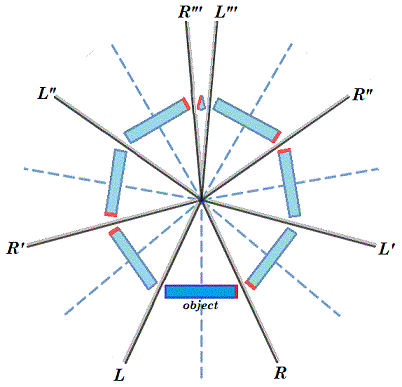The left mirror L is reflected in the right mirror R to L', and the right mirror R is reflected in the left mirror L to R'. Then R is reflected in L' to R'', L is reflected in R' to L'', L is reflected in L' to L''', and R is reflected in R' to R'''.

The result is 6 full images and 1 partial image of the object.

- 1 year, 1 month ago

You took object wide. But if it is point sized two more images will be formed despite of one partial image. I think.

- 1 year, 1 month ago

A point-sized object can be inferred from the diagram. If the point is on the extreme left or right sides of the bar, then there are 7 images, but if the point is on the center of the bar, then there are only 6 images. So a point-sized object placed symmetrically between the two mirrors as described in the question would be like a point on the center of the bar, and would only have 6 images.

- 1 year, 1 month ago

Nice draw , BONUS : draw an arrow along object , to see what image is inverted or not

- 1 year, 1 month ago

To show inversion, I edited the picture so that one edge of the object is red.

- 1 year, 1 month ago

Brilliant then. :)

- 1 year, 1 month ago

The difference between the video David posted and the situation here: the posted video has an object that extends from mirror to mirror, but this problem has a point object.

So when the video shows that 7.2 objects are visible, these are: (a) the original object; (b) six complete images; (c) two partial images of only 1/10th of the object.

Our point object corresponds to the center of the larger object shown in the video. If we look on straight, we can see only six images. But turning our head left or right, we can catch sight of two more.

- 1 year, 1 month ago

In my opinion, 6 images.

My way to deal with these cases is always the same: reflect the world around the mirrors, and reflect again, and again. In the drawing below, left side, you have the direct visible world inside the mirrors, and that one reflected a first time. The green arrow shows where the right mirror puts its first image. The right side shows the full schematic... after repeated reflections over the real mirrors (green), or a single reflection (in this case) over the image of each mirror produced by the previous first reflection operation (yellow).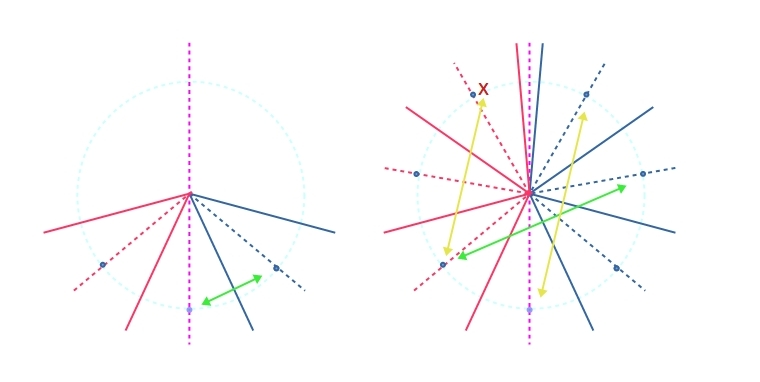This way, you don't need to concern in doing ray reflections... from source to eye-sight, the light can be made to trace a straight line. By mirroring image over the mirrors, your straight line passing through a mirror is equivalent to one that reflects using reflection law. There is however a cardinal rule: The reflections over the right side mirror can only be picked through the right side mirror, and the same for the left mirror. If as an observer, you are in the middle, seeing the images of an object in the middle, by symmetrical mirrors $\alpha$ apart, you'll see only images at $\pm \alpha$, $\pm 2\alpha$, $\pm 3\alpha$, until $\pm 180º$. For instance, in that situation, you cannot see an image at ${\color{#D61F06}X}$, because the light ray doesn't reach the eye through the right mirror (trace a ray and it doesn't cross it... things might be different with a different position for the observer, or if the mirror were semi-transparent and extended behind too).

From this, you can see the number of images seen is:

$2 \left \lfloor{\frac{180}{\alpha}}\right \rfloor$

...when $\alpha$ does not divides 180º, and that number minus 1, when it divides. In our case:

$2 \left \lfloor{\frac{180}{50}}\right \rfloor = 2\times 3=6$

PS.: I think I was too naive in my answer. When you asked how many images, I thought in images I can see... I'm not recalling well the definition of images in more formal contexts, nor will I try to recall it better, because I/you may have learn differently. But I thought I might be more precise in what I was meaning. First, I don't have any problem with what you did. You have a typo in an angle, it should be 100º where you have 110º, but beside that, I think I understand what you did and I have no qualms with that. Second, my 6 image guess is for an observer in the middle of the mirrors (A position). At that position, he'll see the original object and 6 images in the mirrors. But if he changes place, for the B position, he'll see 7 images.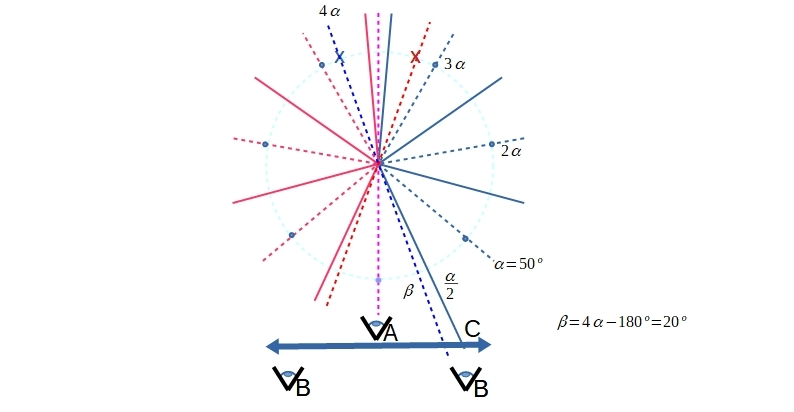Finally, C presents a scenario where we might be aware of 8 images at the same time.

An optical system changes the direction of light, in particular, the light emanated from sources of light we call objects. An image by an optical system is, for me, the point where we would have to put objects to get the same effect if we had not the optical system. This personal definition is probably my source of trouble with your problem, since it leads me to a different number of images depending on the position of an observer. If however we are talking about the full of positions where images can be, then I must agree with the number 8 of your and other answers. The way to prove it is by making a lens big enough to capture all the light between the mirrors, and project it in images after. Then, we'll discover there are 8 images.

By the way, I did the actual experiment with two mirrored doors in my bathroom, making 50º between them. The results agreed with what I said.

- 1 year, 1 month ago

What are you measuring a , 2a , 3a and 180 from. And if you can't see X then you have only for images in your diagram.

Please can you kindly see my image and tell me if i made images wrongly and if not why will i not able to see my last two images. It will help me understand.

- 1 year, 1 month ago

I added some additional information to original comment... just in case to edit it, does not notificate you.

- 1 year, 1 month ago

If you move place of observer , image not move their position, I think you can see or not depending if real ray is intersepted . To prove that is necesary to draw this ray. Is diferent if object moves ( or object with more than one points) , in that case images move too.

- 1 year, 1 month ago

- 1 year, 1 month ago

I know only these people with great minds (excluding me)

Thought to give you some help!

- 1 year, 1 month ago

I have many exams, so I can't help you in this week.

- 1 year, 1 month ago

No problem, All the Best for Exams.

- 1 year, 1 month ago

Thanks!

- 1 year, 1 month ago

Thank you very much. Much appreciated.

And you are too with a beautiful mind.

- 1 year, 1 month ago

Thanks a lot for the kind words @Vikram Karki!

- 1 year, 1 month ago

You added me too and said with great mind. LOL

- 1 year, 1 month ago

I want to say:

1. I haven't studied this in school.
2. I did not understand the problem because of this.
3. (most impotant)I am NOT a great mind.

- 1 year, 1 month ago

Me too, but I tried learning it for the sake of this question

- 1 year, 1 month ago

Yeah, but now you might be able to learn some concepts of reflection when there are two mirrors.

Before School. Exciting Huh?

- 1 year, 1 month ago

Hello Vikram ,

The formula a think is Integer ( 360/50) -1 . That mean on produce 6 images.

I did'n prove that yet , but I will explain way I would do:

Graphicaly all posible ray starting at point has to retorn same point , First ray is that perpendicular at mirror right .Second ray 25º to normal mirror right and reflect to left mirror and for simetry retorn to point . Third ray is 50 º to normal right mirror reflect perpendicular to left mirror and retorn same path . This three ray has 3 more symetric on left mirror , total 6 rays returning to point , that implie 6 images , the ray going from point to vertice not retorn to point then not produice imagen .

PD: going on explanation : We can observe that over ski line (half right) ,angles that alow ray to retorn to point object are 25 , 50 and 75 next will be 100 but is greather than 90 and ray loose . Then number of good angles are Integer ( 90 / (50/2) ) and generalising with any angle between mirrors named "A" , number good angles right side = Integer(180/A) . By simetry , the same for left side ,we multiply by 2 , but if ray with 90º exist , we repeat that (case 180/A = integer) and we must sustract one repetition . Then formula will be : number of images (not including object) = 2 x (integer(180/A) - 1 x (180/A = integer)

Here is drawn only three images , by simmetry on can draw other three . Scale not correct :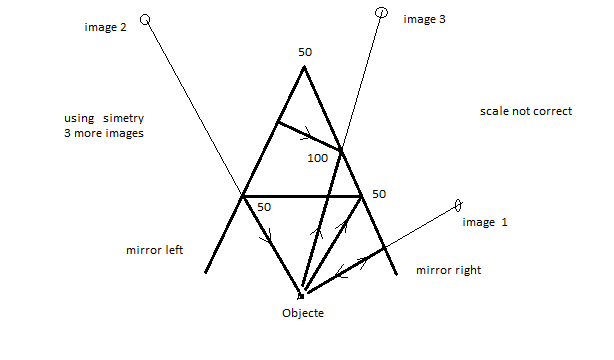- 1 year, 1 month ago

You explained the image formation so, I added explanation of how i made my images and I was wondering if you can read and see if it is right [I think it is].

And I usually make images with paint these ones i just took via phone they automatically saved as png.

- 1 year, 1 month ago

Hello Vikram, while a interpret your draw , I will post mine in TODAY problem for same hours , and after will delete it . Try to see

- 1 year, 1 month ago

Ohh why there And how you made those three images i did not understand.

- 1 year, 1 month ago

I draw only three images for not to fill draw with a lot of lines , other three images are simmetryc of that . Is made with real ray . Distance between object and image no real ( not done with correct scale).

There are practical tools to draw that easily, but without real rays. (you can draw after).

You must considerer ( I supossed and done) , that point of view is located same place than object , that is normal when a person is the object , point of view can move and image keep at place but you must pay attention real rays are not intercepted and keep reflecting on real surface of mirrors.

Formula for angles is n x angle mirrors ( how you do in your draws from origen) , I used angles from object and over horizontal and is half of that . In your case n x angle must keek <= 180º , in mine <= 90º (but is same objective) . Your image Ɪ5 ---> Ɪ7 , Ɪ6 ---> Ɪ8 , violate that (angles greather than 180º for you) .

Your draw is OK for me , except I said images 7 and 8 . A litle lapsus 110º is 100º .

Try to draw over real rays.

- 1 year, 1 month ago

In my explanation Object/virtual object will be will form images until they are behind the mirrors - I used this. Ɪ5 and Ɪ6 are in front of mirror line so they will form images.

I will try to draw with real rays.

And 110 it was a typo :) I saw it after posting.

- 1 year, 1 month ago

• With $50^{\circ}$ as the angle between the two plane mirrors, it is logical to divide that by the total number of degrees $= 360^{\circ}$.
• $\dfrac{360}{50} = 7.2$, a fractional number means a partial image. Thus we will get 7 copies
• But remember that one of them is the original object. Thus we might \boxed{6} and some partial images
Generally speaking, this can be done with any angle $\theta$ instead of $50^{\circ}$. Thus it's formula will be $\dfrac{360}{\theta} - 1$

References: Quora answer, Video as mentioned by @David Vreken

- 1 year, 1 month ago

That means one original object, six images and one 20% of original object( exclude 80 % middle portion of original object).

- 1 year, 1 month ago

Yes, @Mahdi Raza I know this formula and it contradicts with my graph this is why i posted this discussion.

I prefer you to observe my 2nd image or make one of your own to be sure.

[And Frankly speaking - this formula is kind of a trick doesn't always works.

And i don't believe quora I saw a explanation in that site in which a user was dividing 360/50 and his answer was 12.

And video mentioned by David Vreken has a good examples not explanation that formula is correct.]

- 1 year, 1 month ago

And I can prefer a video but it might be 1 and 1/2 hour long.

It is a video of youtube tutor who teaches according to cbse curriculum for JEE.

You might know him Physics Wallah-Alakh Pandey

- 1 year, 1 month ago

Yes I know him, though I don't watch his lectures on youtube very often

- 1 year, 1 month ago

His Videos are good.

- 1 year, 1 month ago

I assume that the object is a point, infinitely small; so you cannot produce fractional images. The only situation in which you get an odd number of images is when the mirror image lies precisely on the line of symmetry. But that cannot be the case here.

It can be shown (I'm sure the video does this, too) that the image points and object lie on a circle, at an arc distance of 50˚ from each other. Thus, if we call the object's position 0˚, and the mirrors cross the circle in ±25˚, then the eight images will be located at ±50˚, ±100˚, ±150˚, ±200˚ (= ±160˚). These last two images will not be reflected further, because they lie on the wrong side of the planes of the mirrors.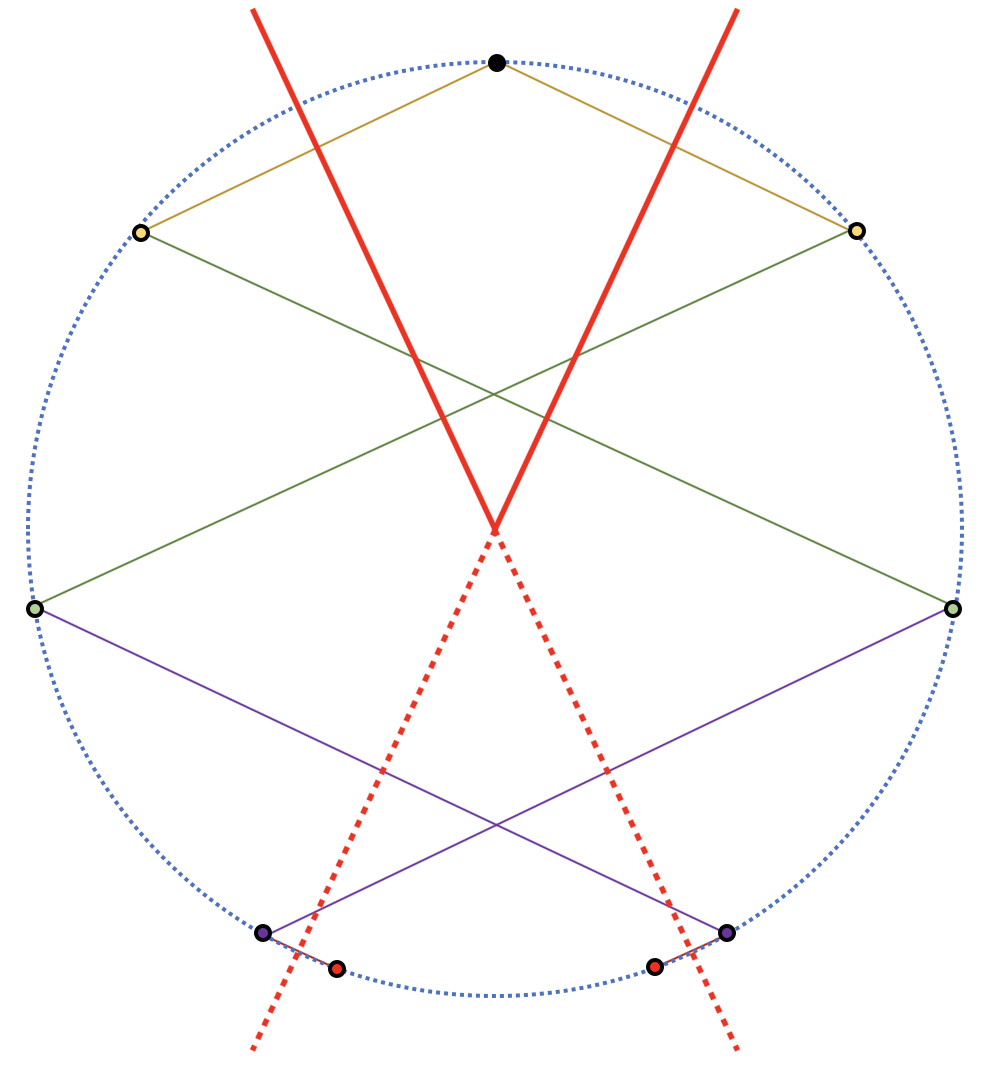In general, if the mirrors form angle $\alpha$, the mirror images will be located at $\pm \alpha, \pm 2\alpha, \pm 3\alpha, \dots$ up to, and including the first value $\pm n\alpha$ where $n\alpha \geq 180˚$. This suggests that the number of images is $2\times \left\lceil\frac{180^\circ}{\alpha}\right\rceil,$ which is equal to $360^\circ/\alpha$ rounded up to the next even integer. In case $\alpha | 180^\circ$, the last image will lie precisely on the line of symmetry and we get an odd number of images, namely $360^\circ/\alpha + 1$.

In this case, we get $360^\circ/50^\circ = 7.2$ which we round up to the next even integer, that is, 8.

- 1 year, 1 month ago

- 1 year, 1 month ago

Yes. As I said, it has to be even because of the obvious symmetry.

- 1 year, 1 month ago

NO, At that time I was talking about general case that it can be both odd or even for ex for 90 it is 3.

- 1 year, 1 month ago

I think last two images ( red points in draw ) , don't exist . It will be there if would exist , but dosn't . Try to draw real ray to produce that.

- 1 year, 1 month ago

He has drawn the ray two small lines joining red points and preceding images.

Can you reason why they don't exist I really want to know.

- 1 year, 1 month ago

Imagen realy exist (virtual in this case) if you can draw real ray from object returning to object after necesary reflection . First 6 images do that , but last two not . Try to do it ( I mean real ray)

- 1 year, 1 month ago

I don't know if i got you right but I am going to make ray diagram for one of the red point images and post here.

- 1 year, 1 month ago

I tryed to post my draw , but at the moment I didn't get ,neither .

- 1 year, 1 month ago

Now i don't know how to post a image in comment.

- 1 year, 1 month ago

I tryed too and diden't get .

- 1 year, 1 month ago

Try creating a note, post a picture, and copy that text and paste it here. That should do the trick!

- 1 year, 1 month ago

The last two can be done too: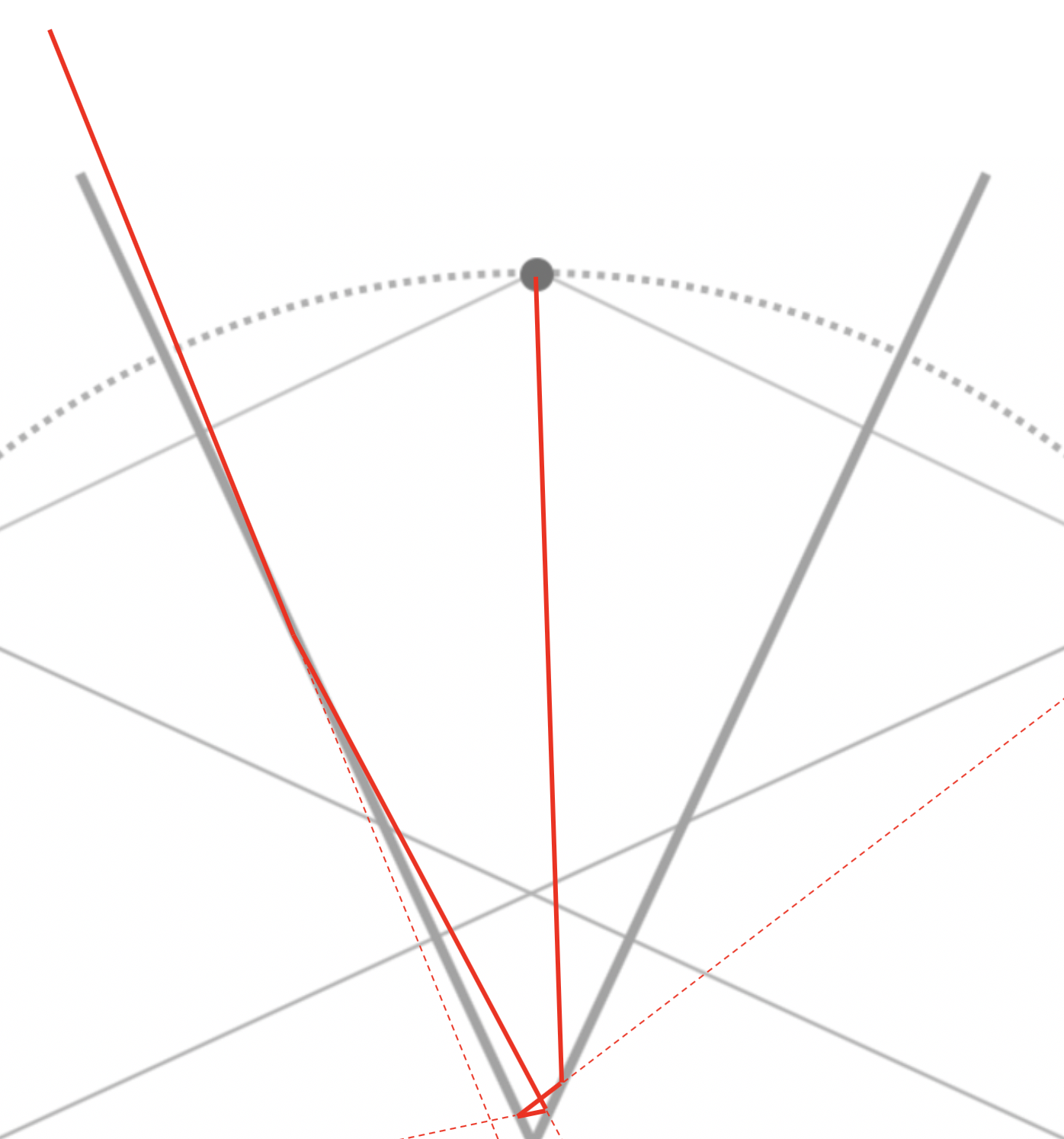- 1 year, 1 month ago

This ray don't retorn to original objet . This image can't be seen from object position. How you post draw?

- 1 year, 1 month ago

Upload in a note, and copy the link(not the URL :D). You don't have to submit the note.

- 1 year, 1 month ago

Rays don't have to go to the original object. The ray must go to the eye of the observer.

- 1 year, 1 month ago

what if observer is at object's place.

- 1 year, 1 month ago

- 1 year, 1 month ago

It shows a light ray that shows in one of the images made by four reflections.

- 1 year, 1 month ago

So, I am right about the solution and formula lies.

Or we have to know more on how to use this formula [360/angle-1]

- 1 year, 1 month ago

Note that he used the ceiling function (upper brackets) which means round $\textit{up}$ to nearest integer

Edit:

Indeed, it is not clear how to use this formula since there are various(right and wrong) explanations

- 1 year, 1 month ago

Yes, but I was talking about the formula everybody else is talking about in their solutions.

- 1 year, 1 month ago

Do you mean even number of images (with repetitions)? There would be an odd number of $\textit{distinct}$ images if the last two happen to overlap

Based on the formula $2 \times \left \lceil \dfrac{180}{\alpha} \right \rceil$

I shall write it as :

$2 \times \left \lceil \dfrac{180}{\alpha} \right \rceil + \left \lceil \dfrac{180}{\alpha} \right \rceil - \left \lfloor \dfrac{180}{\alpha} \right \rfloor -1$

Where :

(floor function $\lfloor \text{ }\rfloor$ )means round $\textit{down}$ to nearest integer

(ceiling function $\lceil \text{ } \rceil$ )means round $\textit{up}$ to nearest integer

The additional part will be (-1 if $\alpha$ is a factor of 180) and (0 otherwise)

This will include both cases. However, it is a bit complicated to write.

- 1 year, 1 month ago

Okay sir Can you explain the part from the formula you provided. I didn't get it.

- 1 year, 1 month ago

Shouldn't the mirrors themselves also be reflected in each other?

- 1 year, 1 month ago

This is what happening when we took a image and consider it a virtual object for other mirror and make another image.

- 1 year, 1 month ago

With your picture, Vikram, I'm having trouble with you using the extended part of the mirror to reflect the points (for example, I3 to I5, I5 to I7, I4 to I6, I6 to I8), because that extended part of the mirror is behind the original mirrors. I think the multiple images have to come from the multiple reflections of the original mirrors in each other.

- 1 year, 1 month ago

Okay, I understand that it might difficult.

We can reflect images using extended mirrors it is valid i have checked it obeys all laws.

why i did that because using real mirror for all formations will turn out to be messy more than it is now.

And difficult too for last two images because they are real close to mirrors. ray diagram will be too messy.

- 1 year, 1 month ago

It think the answer is that eight images are formed.

I am not sure, and I don't know whether I am right or not

I don't know these type of questions

-EDIT-

The formula I used is $\frac{360°}{x°}$ (if and only $\frac{360°}{x°}$ is odd, if it is even, we use $\frac{360°}{x°} - 1$ )

$\frac{360°}{50°} = 7.2$

As seven is odd, and it is approximately near to the answer we got, $7.2$, we need not subtract, and there are in total approximately seven images formed.

It might be safe to say that seven images are formed

- 1 year, 1 month ago

But to be sure and confident i posted so that people with great minds(as you said) can help.

- 1 year, 1 month ago

@Vikram Karki, I gave the answer now; hope you can understand!

- 1 year, 1 month ago

@Vikram Karki, I will post a graphical solution soon...

Stay tuned!

- 1 year, 1 month ago

x is 50

- 1 year, 1 month ago

Hahaha, thanks for mentioning! Didn't read the main question properly!

- 1 year, 1 month ago

actually this is the formula which started all this havoc(kind of) for the solution of this problem.

        A Short story :- I was just putting values in this formula and making graph for the images this is the value where formula contradicted with graph for first time and I literally made graph again and again tens of time actually I have doubt on my graphs until now This is why this Discussion


- 1 year, 1 month ago

I will try to put on a graphical illustration...

That may help

- 1 year, 1 month ago

I think this one is based on this principle

Multiple reflections is equivalent to"reflecting" the real world multiple times. Imagine dividing a circle into portions of 50° each. There would be 7 complete reflections.

Your method is a different. You reflect the same point along the mirror lines as axis of reflections. Thus, you got 8.

I don't know which one is correct. You would reach the same conclusion either way if you use factors of 180° such as 30° . Try angles like 40° first using both methods.

- 1 year, 1 month ago

I do agree dividing circle in portions of given angle works sometimes for eg. for the angles 90,30,even 40. But only

-when object is symmetric to both mirrors

-And for Large angles like 90 and small angles like 30.

Its is kind of a trick and tricks don't always work.

I worked on the angle of 40 the trick worked but just barely what if you increased angle to 45 it works both ways.

But it is not working for 50

- 1 year, 1 month ago

Actually one of them is the original object, so maybe 6 is the answer

- 1 year, 1 month ago

I only understand the first pair of images, don't know how you progress after that.

- 1 year, 1 month ago

Actually I did the same as first.

Look carefully.

- 1 year, 1 month ago

I would argue that the number of images should be even, because of symmetry.

- 1 year, 1 month ago

Not sure, but that depends on the angle between the two plane mirrors

- 1 year, 1 month ago

Not truly,

• Sometimes no. of images comes to be odd as half of image is produced by one mirror and half of image is produced by other.

• Sometimes two images overlap causing decrease in one image.

What i want you to do is observe my 2nd image it is the image formation on a cartesian plane. I had used simple reflection laws to form that and no. of images comes out to be 8.

[I have added explanation in the note.]

- 1 year, 1 month ago

Your picture is incorrect. The second image should be $100^{\circ}$

- 1 year, 1 month ago

who are you saying to?

- 1 year, 1 month ago

Because 90+10=100 and 90+10 can't be 110.

- 1 year, 1 month ago

But Vikram's drawing has 90 + 10 = 100˚, as it should be.

- 1 year, 1 month ago

Yes, but he wrote that incorrectly.

- 1 year, 1 month ago

You're quite a observer.

- 1 year, 1 month ago

I never noticed that. God gifted you with a sharp eye @Páll Márton

- 1 year, 1 month ago

I thought no one will notice that

- 1 year, 1 month ago

Oh yes, talking about the small image in corner of 2nd.

- 1 year, 1 month ago

In my Physics Online Class of this year, I was told the same formula given by @David Vreken and @Mahdi Raza, but it is also told that the no. of images is always odd, I don't know why.

- 1 year, 1 month ago

Not always, Sometimes two images overlap but formula counts both images and we have to remove that ourselves. for ex: if angle is 90 degrees 360/90 = 4 (even) ==> subtract 1 = 3 in this case last two images overlap(you can see image in my post above).

In my school we are not taught how to use this formula properly this is why I don't always use it.(I sometimes do)

- 1 year, 1 month ago